Question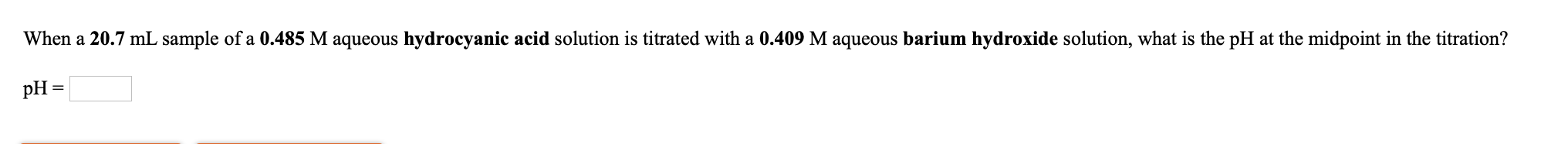In an acid base titration, the mid point is that point where exactly half the amount of acids present by the base added.

Now here we have the weak acid Hydrocyanic acid (HCN). Now when we add a strong base like Ba(OH)2, then at mid point, half of he aicds have been converted to its conjugate base.

i.e

HCN (weak acid) + Ba(OH)2 ----------> CN- (conjugate base) + H2O

so we can say at this point, exactly equal amount of HCN and CN- are present. Now since in this both the weak acid and its conjugate base are present, this solution becomes a buffer. And the pH of buffer is calculated by Henderson- Hasselblach equation-

pH = pKa + log [conjugate base] / [weak acid]

Now for HCN, pKa = 9.2

So we can write-

pH = 9.2 + log [CN-] / [HCN]

Now since we found at mid point, [CN-] = [HCN]

So

pH = 9.2 + log 1

= 9.2 + 0

= 9.2

Or simply we can say, at mid point of ttration, pH of the solution = pKa of the acid

#### Earn Coins

Coins can be redeemed for fabulous gifts.

Similar Homework Help Questions
• ### this question. When a 21.0 mL sample of a 0.411 M aqueous hydrocyanic acid solution is...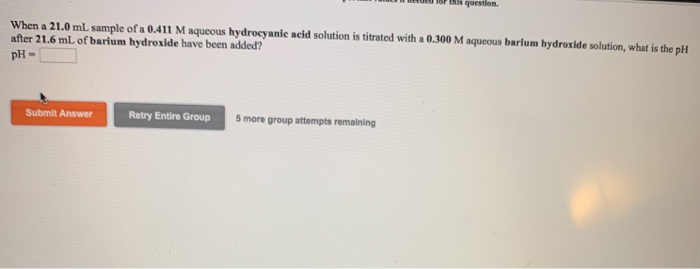this question. When a 21.0 mL sample of a 0.411 M aqueous hydrocyanic acid solution is titrated with a 0.300 M aqueous barium hydroxide solution, what is the pH after 21.6 mL of barium hydroxide have been added? PH Submit Answer Retry Entire Group 5 more group attempts remaining What is the pH at the equivalence point in the titration of a 23.3 ml sample of a 0.347 M aqueous hypochlorous acid solution with a 0.395 M aqueous barium hydroxide...

• ### When a 21.4 mL sample of a 0.311 M aqueous hydrocyanic acid solution is titrated with...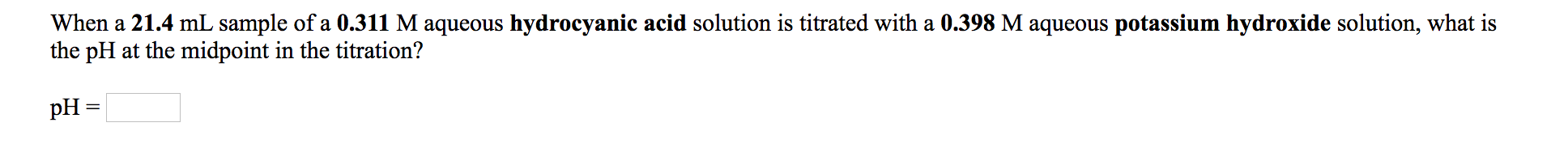When a 21.4 mL sample of a 0.311 M aqueous hydrocyanic acid solution is titrated with a 0.398 M aqueous potassium hydroxide solution, what is the pH at the midpoint in the titration? pH = A 45.3 mL sample of a 0.370 M aqueous acetic acid solution is titrated with a 0.424 M aqueous potassium hydroxide solution. What is the pH after 23.1 mL of base have been added? pH =

• ### 1. When a 21.5 mL sample of a 0.329 M aqueous hypochlorous acid solution is titrated...

1. When a 21.5 mL sample of a 0.329 M aqueous hypochlorous acid solution is titrated with a 0.456 M aqueous potassium hydroxide solution, what is the pH after 23.3 mL of potassium hydroxide have been added? pH = 2. When a 16.0 mL sample of a 0.404 M aqueous hydrocyanic acid solution is titrated with a 0.418 M aqueous barium hydroxide solution, what is the pH at the midpoint in the titration? pH =

• ### When a 17.3 mL sample of a 0.447 M aqueous hydrocyanic acid solution is titrated with...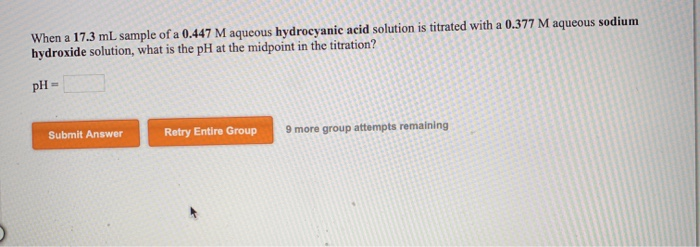When a 17.3 mL sample of a 0.447 M aqueous hydrocyanic acid solution is titrated with a 0.377 M aqueous sodium hydroxide solution, what is the pH at the midpoint in the titration? pH = Submit Answer Retry Entire Group 9 more group attempts remaining A 36.4 mL sample of a 0.391 M aqueous hydrofluoric acid solution is titrated with a 0.250 M aqueous sodium hydroxide solution. What is the pH after 17.9 mL of base have been added? pH...

• ### When a 15.6 mL sample of a 0.328 M aqueous hydrocyanic acid solution is titrated with...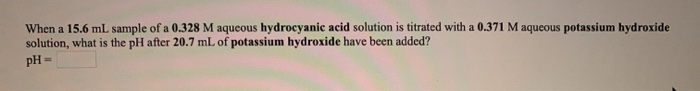When a 15.6 mL sample of a 0.328 M aqueous hydrocyanic acid solution is titrated with a 0.371 M aqueous potassium hydroxide solution, what is the pH after 20.7 mL of potassium hydroxide have been added? pH

• ### When a 23.8 mL sample of a 0.443 M aqueous hydrofluorie acid solution is titrated with...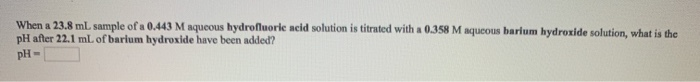When a 23.8 mL sample of a 0.443 M aqueous hydrofluorie acid solution is titrated with a 0.358 M aqueous barium hydroxide solution, what is the pH after 22.1 mL of barium hydroxide have been added? pH- What is the pH at the equivalence point in the titration of a 19.7 mL sample of a 0.376 M aqueous nitrous acid solution with a 0.447 M aqueous sodium hydroxide solution? pH- When a 20.1 mL sample of a 0.417 M aqueous...

• ### When a 24.9 mL sample of a 0.438 M aqueous acetic acid solution is titrated with...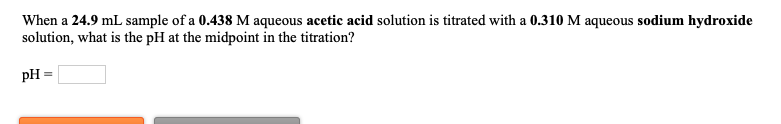When a 24.9 mL sample of a 0.438 M aqueous acetic acid solution is titrated with a 0.310 M aqueous sodium hydroxide solution, what is the pH at the midpoint in the titration? pH = What is the pH at the equivalence point in the titration of a 17.5 mL sample of a 0.380 M aqueous hydrofluoric acid solution with a 0.379 M aqueous barium hydroxide solution? PH = 3.14

• ### When a 17.2 mL sample of a 0.324 M aqueous hypochlorous acid solution is titrated with...

When a 17.2 mL sample of a 0.324 M aqueous hypochlorous acid solution is titrated with a 0.319 M aqueous barium hydroxide solution, (1) What is the pH at the midpoint in the titration? (2) What is the pH at the equivalence point of the titration? (3) What is the pH after 13.1 mL of barium hydroxide have been added?

• ### When a 23.7 mL sample of a 0.324 M aqueous hypochlorous acid solution is titrated with...

When a 23.7 mL sample of a 0.324 M aqueous hypochlorous acid solution is titrated with a 0.328 M aqueous barium hydroxide solution, what is the pH at the midpoint in the titration? pH =

• ### When a 16.0 mL sample of a 0.378 M aqueous hypochlorous acid solution is titrated with...

When a 16.0 mL sample of a 0.378 M aqueous hypochlorous acid solution is titrated with a 0.357 M aqueous barium hydroxide solution, what is the pH at the midpoint in the titration?